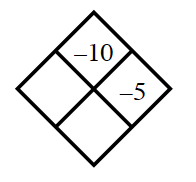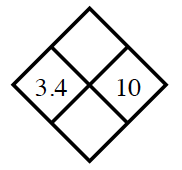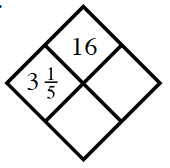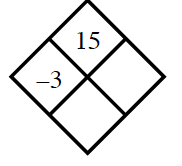### Home > ACC6 > Chapter 3 Unit 11 > Lesson CC2: 3.3.3 > Problem3-123

3-123.

Copy and complete each of the Diamond Problems below. The pattern used in the Diamond Problems is shown at right.1.What do you multiply $-5$ by to get $-10$?

Don't forget to find the sum!

1.Using your addition and multiplication skills, try finding the sum and product of the numbers above.

Product: $34$
Sum: $13.4$

1.Using division, first try to find the $y$-value, the number in the right of the diamond. Do your best!

1.Follow the same method you used for part (c).

Right number: $-5$
Bottom number: $-8$